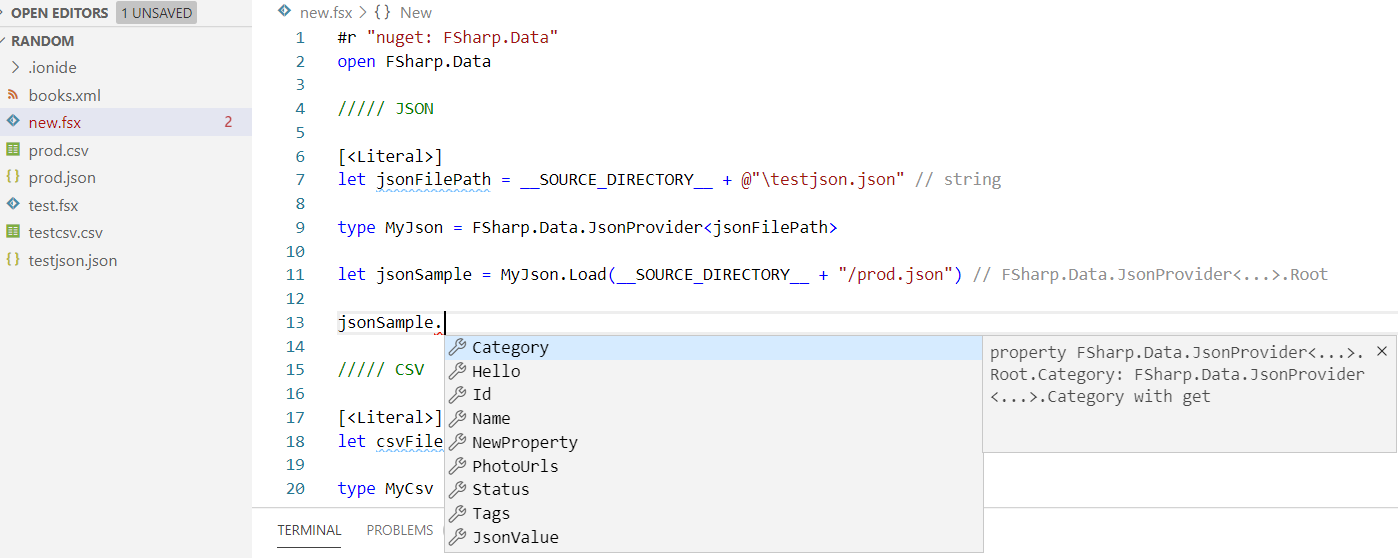# F#5 like a Pythonista on steroidsF#5 in action in vscode + ionide

# Getting Started

• install latest dotnet SDK for NET5
• install vscode and add all ionide extensions
• install Microsoft.dotnet-interactive as a global tool
`mkdir fsx && cd fsx; touch new.fsx; code .`

# VSCode and IONIDE

Ionide is a great F# cross platform IDE plug-in for VScode, it works pretty good for F# scripting, sometimes might need you to clear cache or restart vscode though.

# A function in Python

`def sum(a,b):    return a + b`

# The same function in F#

`let sum a b = a + b // or quicker, let sum = (+)`

# A class in Python

`class Dog:    species = "Canis familiaris"    def __init__(self, name, age):        self.name = name        self.age = age    def description(self):        return f"{self.name} is {self.age} years old"    def speak(self, sound):        return f"{self.name} says {sound}"`

# A class in F#

`type Dog(name,age) =  member val Description =      \$"{name} is {age} years old"  member this.Speak(sound) =           \$"{name} says {sound}"  member val Species = "canis familiaris"`

# Manipulating Sequences (*)

F#: with the pipe operator |> and List module functions:

`let sumOfEvenNumbersFromOneTo100Adding1 =   [1..100]   |> List.filter (fun x -> x % 2 = 0)   |> List.map (fun x -> x + 1)   |> List.sum //2600`
`sample2 = range(1,101) # 1..100filteredList = list(filter(lambda x : x % 2 == 0, sample2))addOneList = list(map(lambda x : x + 1 , filteredList))total = sum(addOneList) # 2600`

# List Comprehensions (*)

F#: list comprehensions are a powerful construct enabled by computation expressions, so regular F# code is valid within a list comprehension!

`let listComprehension =   [      for x in [1..100] do         let z = "hello" // just to show you can!         if x % 2 = 0 then              yield x + 1 // value is returned whenever we want   ]`
`list_comprehension =    [          x + 1 # value is always returned at the top       for x in range(1,101) # 1..100       if x % 2 == 0   ]`

# Reading a JSON file

As an example task, we want to read some fields in a json file, so parse it and display the captured value on the console.

# In Python

`import jsonwith open('test.json', 'r') as myfile:data = myfile.read()obj = json.loads(data)print(obj["hello"])`

# In F# with JsonParser

`#r "nuget: FSharp.Data"open FSharp.Dataopen FSharp.Data.JsonExtensions // ? oplet result =    (__SOURCE_DIRECTORY__ + "/test.json")   |> System.IO.File.ReadAllText   |> JsonValue.Parseprintfn \$"{result?Hello}"`

# In F# with Type Providers

`#r "nuget: FSharp.Data"open FSharp.Data[<Literal>]let jsonFilePath = __SOURCE_DIRECTORY__ + @"/test.json"type MyJson = JsonProvider<jsonFilePath>let result = MyJson.Load(__SOURCE_DIRECTORY__ + "/prod.json")printfn \$"{result.Hello}" //strongly typed!`

# Reading a CSV file

`Name,Surname,AgeJohn,Red,33Mike,Bianchi,55...`

# Python

`import csvwith open('test.csv') as csv_file:    csv_reader = csv.reader(csv_file, delimiter=',')        for row in csv_reader:         print(row) //Name`

# F#

`#r "nuget: FSharp.Data"open FSharp.Data[<Literal>]let csvPath = __SOURCE_DIRECTORY__ + "/test.csv"type MyCsv = CsvProvider<csvPath, ",">for row in MyCsv.GetSample().Rows do   printfn \$"{row.Name}" // first column header = Name`

# Python

`import plotly.express as pxdf = list(range(1, 11)) //1...10fig = px.line(df)fig.show()`

# F#

`#r "nuget: XPlot.Plotly"open XPlot.Plotly[ 1 .. 10 ] |> Chart.Line |> Chart.Show`

# Python

`from flask import Flaskapp = Flask(__name__)@app.route('/')def index():    return 'Hello World!'app.run(host='0.0.0.0', port=81)`

# F#

`#r "nuget: Suave"open Suave  startWebServer defaultConfig (Successful.OK "Hello World!")`

--

--